# The QLIM Procedure

### Selection Models

Subsections:

In sample selection models, one or several dependent variables are observed when another variable takes certain values. For example, the standard Heckman selection model can be defined as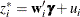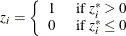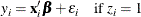where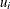and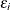are jointly normal with 0 mean, standard deviations of 1 and, respectively, and correlation of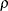. Selection is based on the variable z, and y is observed when z has a value of 1. Least squares regression that uses the observed data of y produces inconsistent estimates of. The maximum likelihood method is used to estimate selection models. It is also possible to estimate these models by using Heckman’s method, which is more computationally efficient. But it can be shown that the resulting estimates, although consistent, are not asymptotically efficient under a normality assumption. Moreover, this method often violates the constraint on the correlation coefficient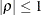.

The log-likelihood function of the Heckman selection model is written as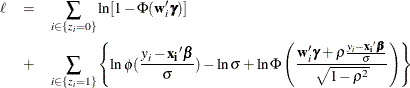The selection can be based on only one variable, but the selection can lead to several variables. For example, selection is based on the variable z in the following switching regression model: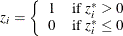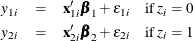If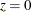, then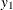is observed. If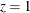, then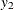is observed. Because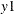and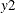are never observed at the same time, the correlation betweenandcannot be estimated. Only the correlation between z andand the correlation between z andcan be estimated. This estimation uses the maximum likelihood method.

A brief example of the SAS statements for this model can be found in Sample Selection Model.

The Heckman selection model can include censoring or truncation. For a brief example of the SAS statements for these models see Sample Selection Model with Truncation and Censoring. The following example shows a variablethat is censored from below at zero: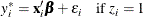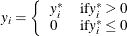In this case, the log-likelihood function of the Heckman selection model needs to be modified as follows to include the censored region: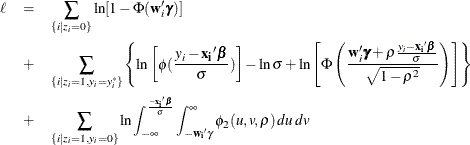In caseis truncated from below at 0 instead of censored, the likelihood function can be written as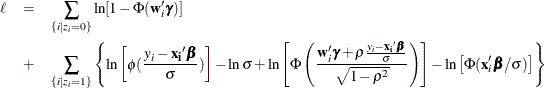#### Heckman’s Two-Step Selection Method

Sample selection bias arises from nonrandom selection of the sample from the population. A classic example is using a sample of market wages for working women to estimate female labor supply function. This sample is nonrandom because it includes only the wages of women whose market wage exceeds their home wage at zero hours of work.

A simple selection model can be written as the latent modelwhereandare jointly normal with 0 mean, standard deviations of 1 and, respectively, and correlation of. The dependent variable(wage) is observed if the latent variable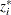(the difference between market wage and reservation wage) is positive or if the indicator variable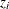(labor force participation) is 1.

The model of interest that applies to the observations in the selected sample can be written as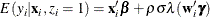where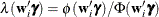. Hence, the following regression equation is valid for the observations for which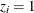: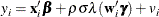Therefore, estimates ofthat are obtained from the OLS regression of y onby using the selected sample (that is, the sample for which) suffer from omitted variable bias if selection bias is really the case. Although maximum likelihood estimation ofis consistent and efficient, Heckman’s two-step method is more frequently used. Heckman’s two-step method can be requested by specifying the HECKIT option of the QLIM statement.

Heckman’s two-step method is as follows:

1. Obtain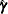, the estimate of the parameters of the probability that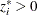, by using regressors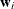and the binary dependent variableby probit analysis for the full sample. Compute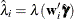.

2. Obtain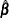and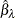, the estimates ofand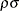, by least squares regression of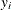on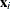and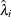by using observations on the selected subsample.

The standard least squares estimators of the population varianceand the variances of the estimated coefficients are incorrect. To test hypotheses, the correct ones need to be calculated. An estimator ofis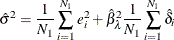where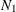is the selected subsample size,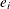is the residual for the ith observation obtained from step 2, and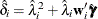. Let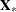be an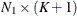matrix with ith row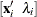, and define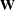similarly with ith row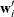. Then the estimator of the asymptotic covariance of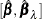is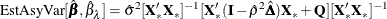where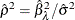,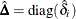, andwhere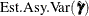is the estimator of the asymptotic covariance of the probit coefficients that are obtained in step 1. When you specify the HECKIT option, PROC QLIM uses a numerical estimated asymptotic variance.

When the HECKIT option is specified, PROC QLIM reports the corrected standard errors for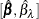automatically. However, if you need the conventional OLS standard errors, you can specify the HECKIT(UNCORRECTED) option.

In the selected regression model, when the coefficient of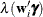is 0, you do not need Heckman’s two-step estimation method; a simple regression of y onproduces consistent estimates for, and the OLS standard errors are correct. Thus, a standard t test on(which uses the estimate from step 2 and the uncorrected standard errors) is a valid test of the null hypothesis of no selection bias.

Although Heckman’s two-step method uses the OLS method in the second stage, you can request the ML method by specifying the HECKIT(SECONDSTAGE=ML) option. When the second-stage method is the ML method, the model forcan be nonlinear.